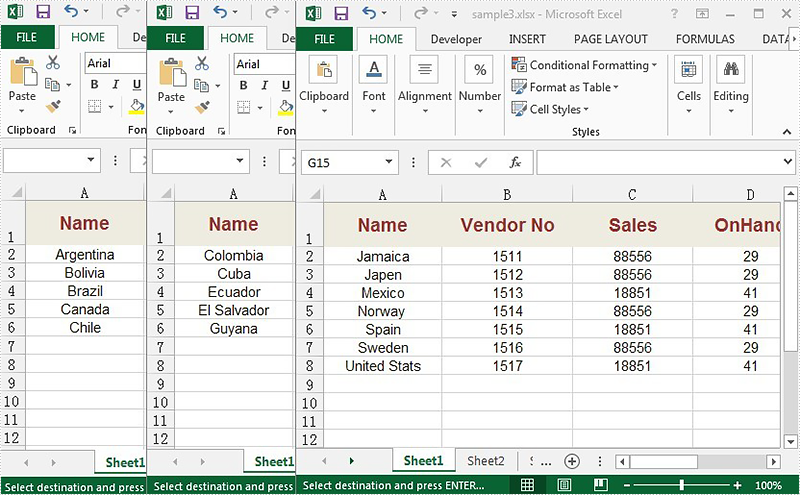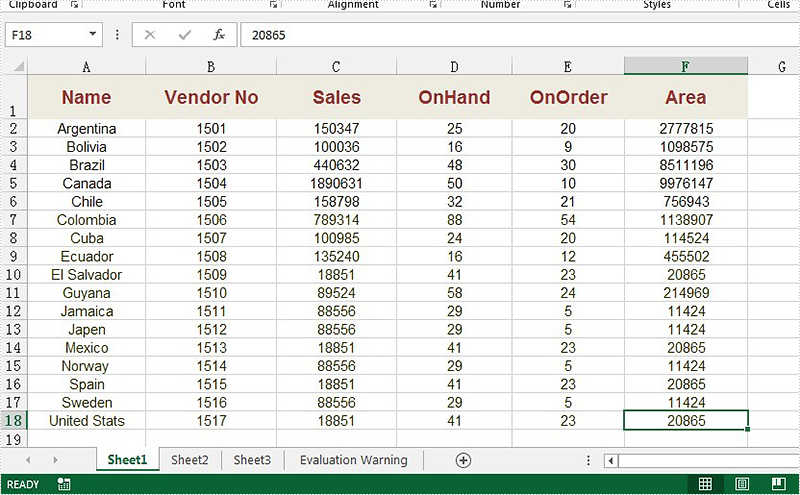# How to merge several Excel worksheets into a single Excel worksheet

We have already demonstrated how to merge multiple workbooks into a single workbook. This example shows how to merge multiple worksheets from different workbooks into a single worksheet by using the method Worksheet.Range.Copy(); offered by Spire.XLS.

Here comes to the details of how to merge Excel worksheets into one worksheet. Firstly, view the source files for different worksheets from different Excel workbooks:Step 1: Create a new workbook and load from file.

```Workbook MerBook = new Workbook();
```

Step 2: Get the first worksheet from workbook.

```Worksheet MerSheet = MerBook.Worksheets;
```

Step 3: Create the second workbook and load from file.

```Workbook SouBook1 = new Workbook();
```

Step 4: Define the LastRow and LastColumn for the first worksheet in the second workbook.

```int a = SouBook1.Worksheets.LastRow;
int b = SouBook1.Worksheets.LastColumn;
```

Step 5: Copy the data from the defined range on the second workbook.

```SouBook1.Worksheets.Range[2, 1, a, b].Copy(MerSheet.Range[MerSheet.LastRow + 1, 1, a + MerSheet.LastRow, b]);
```

Step 6: Save the document to file.

```MerBook.SaveToFile("reslut.xlsx", ExcelVersion.Version2010);
```

Effective screenshot:Full codes:

```using Spire.Xls;
namespace MergeExcel
{
class Program
{

static void Main(string[] args)
{
{
Workbook MerBook = new Workbook();
Worksheet MerSheet = MerBook.Worksheets;

Workbook SouBook1 = new Workbook();
int a = SouBook1.Worksheets.LastRow;
int b = SouBook1.Worksheets.LastColumn;
SouBook1.Worksheets.Range[2, 1, a, b].Copy(MerSheet.Range[MerSheet.LastRow + 1, 1, a + MerSheet.LastRow, b]);

Workbook SouBook2 = new Workbook();
int c = SouBook2.Worksheets.LastRow;
int d = SouBook2.Worksheets.LastColumn;
SouBook2.Worksheets.Range[2, 1, c, d].Copy(MerSheet.Range[MerSheet.LastRow + 1, 1, c + MerSheet.LastRow, d]);

MerBook.SaveToFile("reslut.xlsx", ExcelVersion.Version2010);

}

}

}
}
```